• 六种三角函数
千次阅读
2020-03-27 19:22:54

# 三角函数简介

## 描述

简要描述六种三角函数及其图像

### 正弦–sin x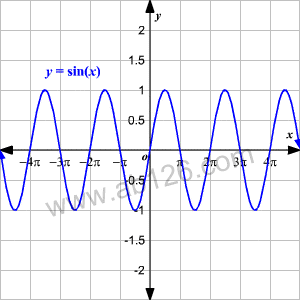### 余弦–cos x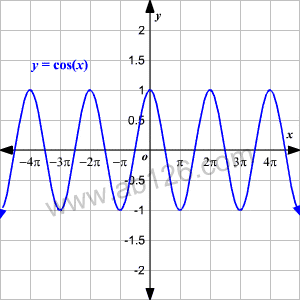### 正切–tan x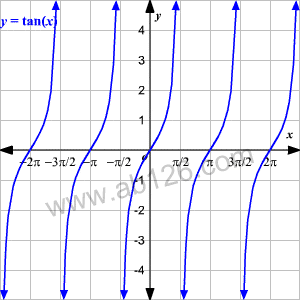### 正割–sec x

• sec x = 1 / cos x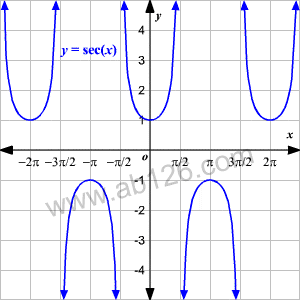### 余割–csc x

• csc x = 1 / sin x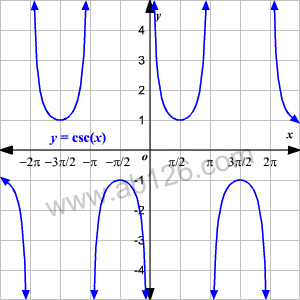### 余切–cot x

• cot x = cos x / sin x = 1 / tan x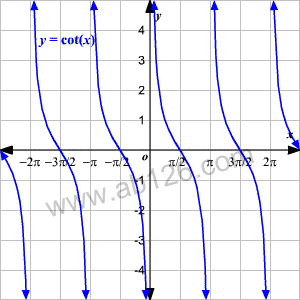更多相关内容
• ## 三角函数

千次阅读 2020-10-31 12:48:51
三角函数分为六种类型 1.正弦函数 y=sinx 性质： x∈R -1<y<1 有界函数，即在-1和1之间活动 周期性为T=2π，2π为一个周期，N个周期组成了连续性 奇偶性 奇函数 注：奇函数远点对称，偶函数y轴对称 ...

三角函数分为六种类型
1.正弦函数
y=sinx性质：
x∈R
-1<y<1 有界函数，即在-1和1之间活动
周期性为T=2π，2π为一个周期，N个周期组成了连续性
奇偶性 奇函数
注：奇函数远点对称，偶函数y轴对称
三边关系  sinx = 对边：斜边


2.余弦函数(正弦函数右移或左移π/2即余弦函数)
y=cosx性质：
x∈R
1>y>-1有界函数
周期性：T=2π
偶函数（对y轴对称）
三边关系  cosx=邻边：斜边


3.正切函数
y=tanx性质：
x≠kπ  k∈Z（Z是整数集合）
y∈R无界函数
周期性 T=π
奇偶性  奇函数
单调性  增函数
三边关系： tanx= 对边：邻边


4.余切函数
y=cotx 此函数为正切函数先对称在评议π/2的图像性质：
x≠kπ k∈Z
y∈R  无界函数
周期性  T=π
奇偶性 奇函数
单调性 减函数
三边关系：cotx=tanx倒过来



5.正割函数
y= secx
6.余割函数
y=cscx

==========================================

## 这6个三角函数之间的关系

6卦图表示## 倒三角平方和公式

即倒三角的两个上面的角的平方和==下面角的²
该图中共有三个倒三角：
分别是sinx cosx和1    tanx 1 和secx   1cotx 和cecx
根据公式可得到：
sinx^2 + cos^2=1
tanx^2 + 1 = secx^2
1 + cotx^2 = cscx^2


## 邻点积公式

六挂图中，任意一个顶点是相邻的两个顶点的积
共有六个顶点（每个三角函数都是一个顶点）

sinx=cosx*tanx
cosx=sinx*cotx
cotx=cosx*cscx
cscx=cotx*secx
secx =cscx*tanx
tanx=sinx+secx


## 二倍角公式

二倍角公式可以延伸出降幂公式
先说一下二倍角公式：
cos2α = 2cos²*α-1
通过运算可以降幂
cos²=cos2+1/2

## 特殊角的三角函数

角度：
0°
30°
45°
60°
90°
弧度：180°==π
0°==0
30°==π/6
45°==π/4
60°==π/3
90°==π/2## 三角函数的符号（±）

正三角函数： 第一象限全为正 二正三切四余弦（正弦 正切 余弦）

## 诱导公式

目的：解决非特殊三角函数【把非特殊变成特殊角度来计算】

公式：
sin角度 = sin(特殊角±k*π/n)  π==180°这里就是将一个角度转换成特殊角+可整除90°的整数
一句话：拆分成可以计算的特殊角
例如：210° == 30°+180°
注意事项：
奇变偶不变，符号看象限 奇偶代表k的值
变和不变是三角函数的名称（sin cos）
符号看象限 就是三角函数的符号公式
符号 原三角函数的原角度的符号（第一象限全为正 二正三切四余弦（正弦 正切 余弦））

例如：sin210°
首先拆分成  sin(30°+2*90°)
2为偶数，函数仍然是sin
看原角度，sin210°在第三象限，而第三象限是正切是是正的，其他全是-的，即这里的符号是-的
可得：-sin30°
==    -根号1/2

展开全文• ## 三角函数公式和图像大全

万次阅读 多人点赞 2021-04-07 12:01:05
三角函数的图形 反三角函数的图形 各三角函数值在各象限的符号 三角函数的性质 反三角函数的性质 三角函数公式 两角和公式 倍角公式 三倍角公式 半角公式 和差化积 积...

初等函数的图形

幂函数的图形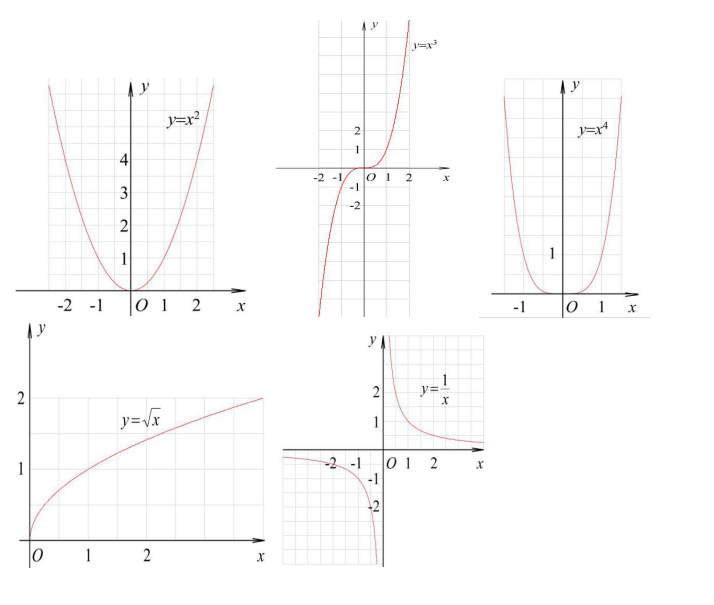指数函数的图形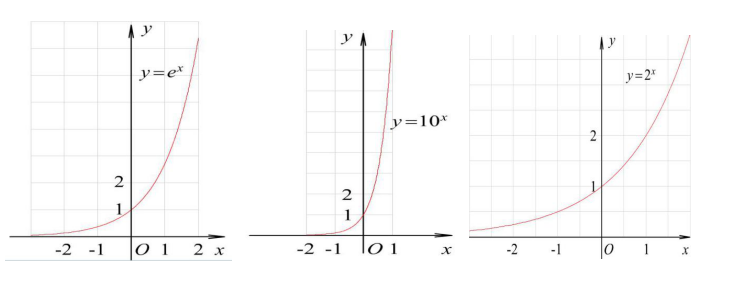对数函数的图形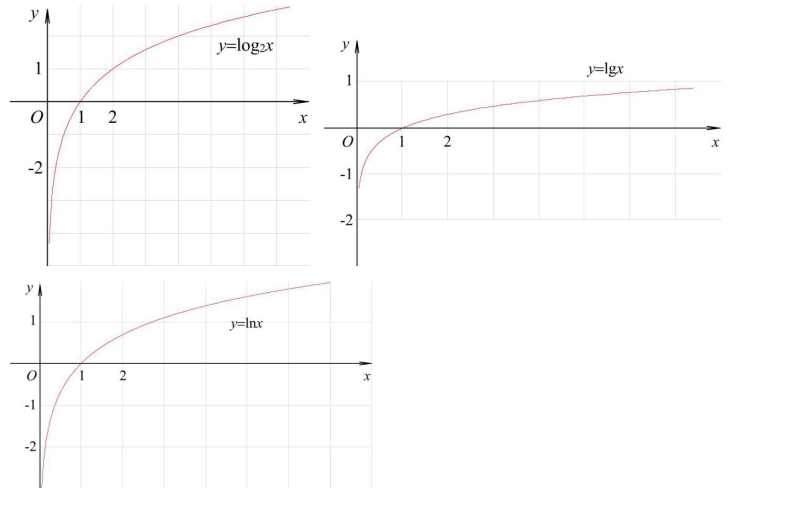三角函数的图形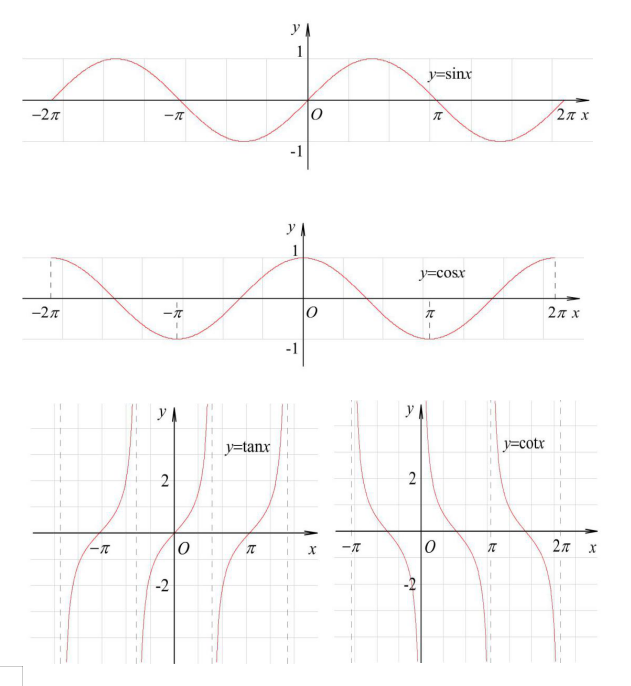反三角函数的图形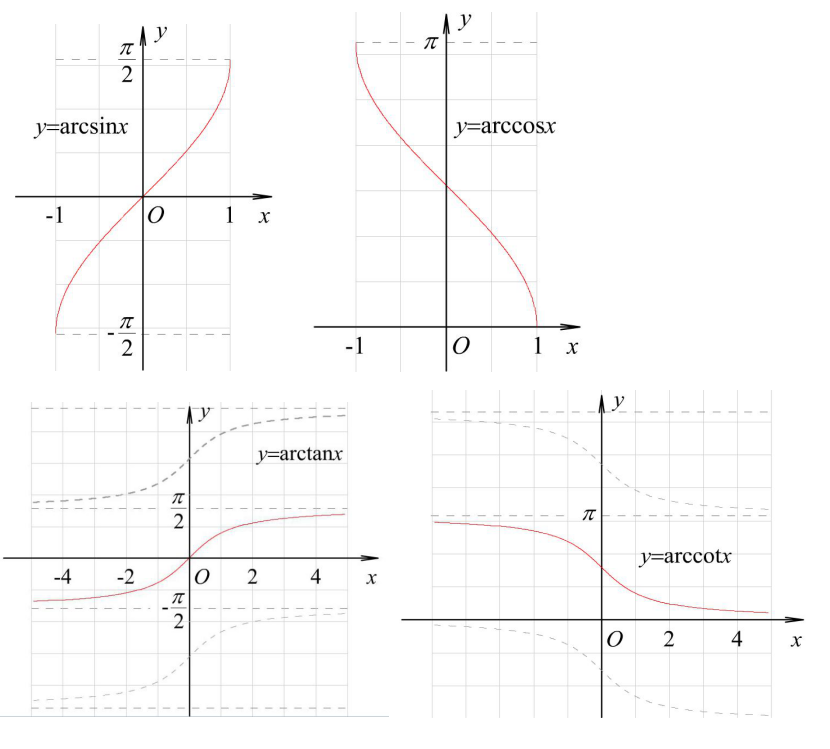各三角函数值在各象限的符号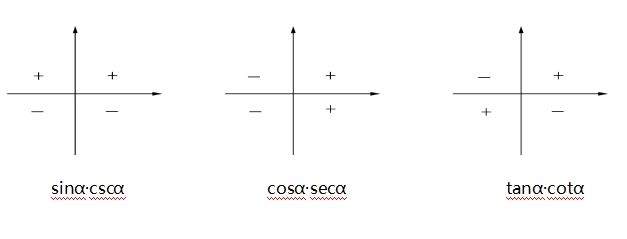三角函数的性质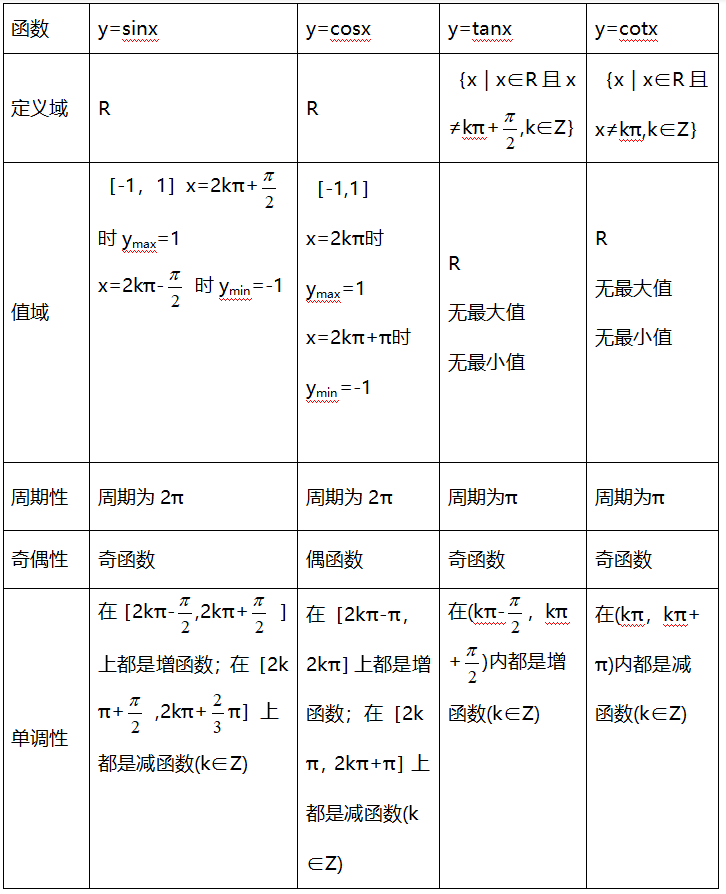反三角函数的性质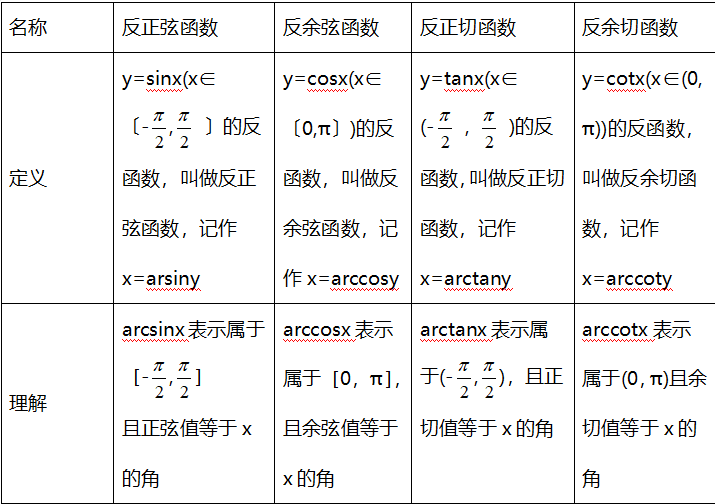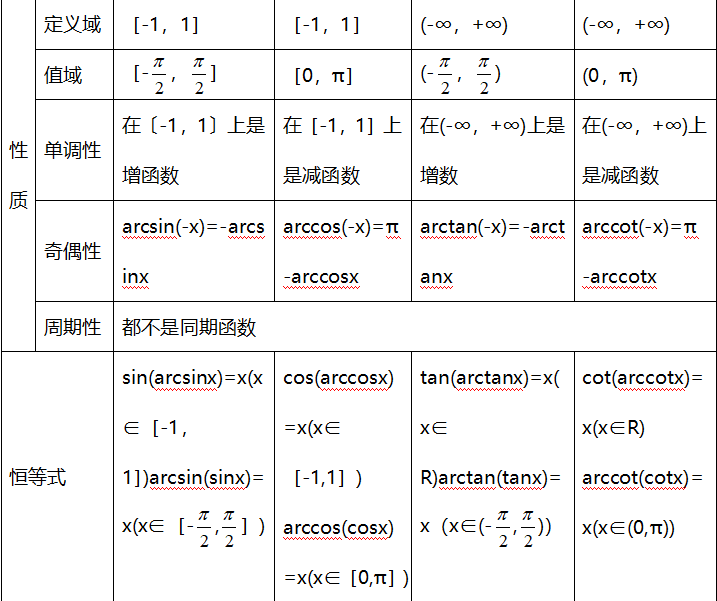三角函数公式

两角和公式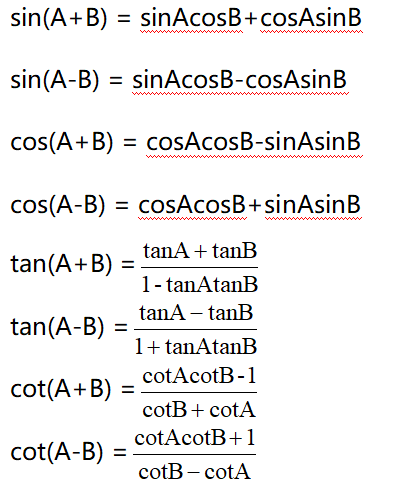倍角公式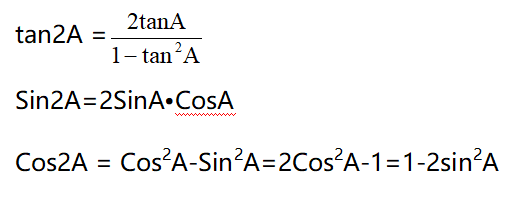三倍角公式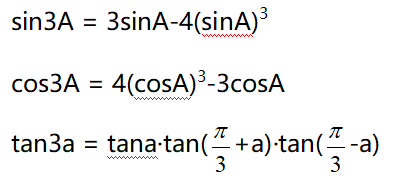半角公式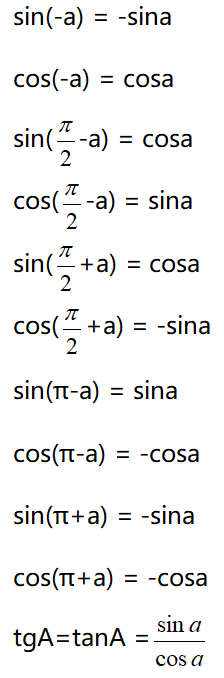和差化积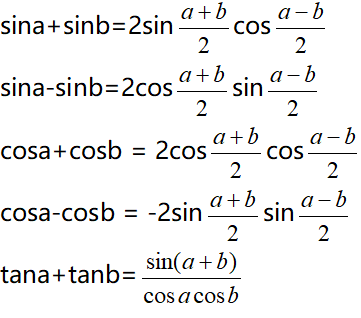积化和差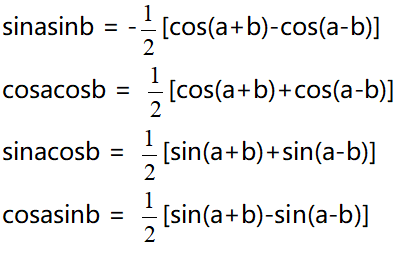诱导公式万能公式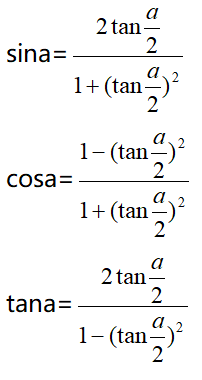其它公式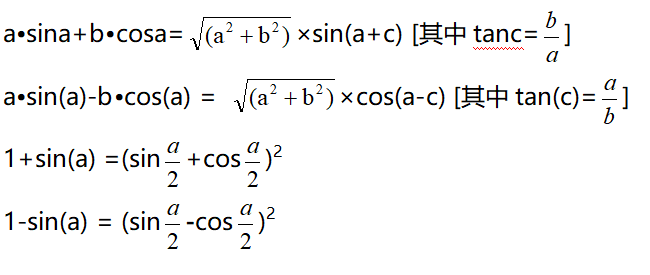其他非重点三角函数双曲函数公式一

设α为任意角，终边相同的角的同一三角函数的值相等：

sin（2kπ＋α）= sinα

cos（2kπ＋α）= cosα

tan（2kπ＋α）= tanα

cot（2kπ＋α）= cotα

公式二

设α为任意角，π+α的三角函数值与α的三角函数值之间的关系：

sin（π＋α）= -sinα

cos（π＋α）= -cosα

tan（π＋α）= tanα

cot（π＋α）= cotα

公式三

任意角α与 -α的三角函数值之间的关系：

sin（-α）= -sinα

cos（-α）= cosα

tan（-α）= -tanα

cot（-α）= -cotα

公式四

利用公式二和公式三可以得到π-α与α的三角函数值之间的关系：

sin（π-α）= sinα

cos（π-α）= -cosα

tan（π-α）= -tanα

cot（π-α）= -cotα

公式五

利用公式-和公式三可以得到2π-α与α的三角函数值之间的关系：

sin（2π-α）= -sinα

cos（2π-α）= cosα

tan（2π-α）= -tanα

cot（2π-α）= -cotα

公式六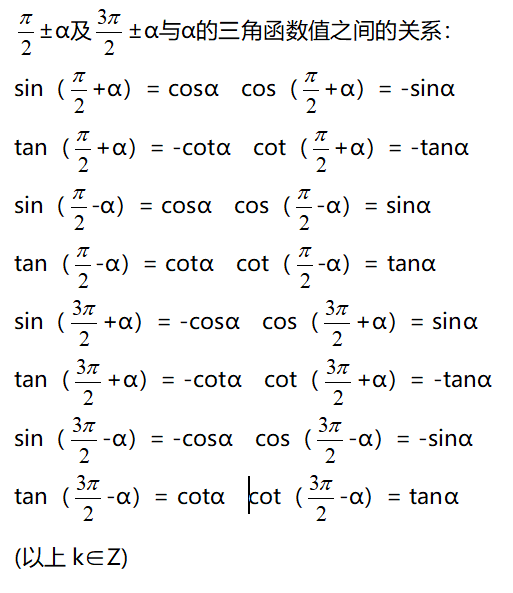这个物理常用公式我费了半天的劲才输进来,希望对大家有用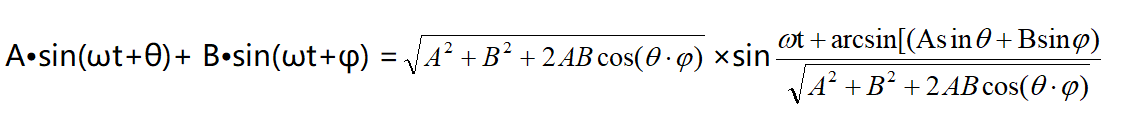三角函数公式证明（全部）

公式表达式

乘法与因式分解

a2-b2=(a+b)(a-b) a3+b3=(a+b)(a2-ab+b2) a3-b3=(a-b)(a2+ab+b2)

三角不等式

|a+b|≤|a|+|b|

|a-b|≤|a|+|b|

|a|≤b<=>-b≤a≤b

|a-b|≥|a|-|b|

-|a|≤a≤|a|

一元二次方程的解

-b+√(b2-4ac)/2a -b-b+√(b2-4ac)/2a

根与系数的关系

X1+X2=-b/a

X1*X2=c/a

注：韦达定理

判别式 b2-4a=0 注：方程有相等的两实根

b2-4ac>0 注：方程有一个实根

b2-4ac<0 注：方程有共轭复数根

三角函数公式

两角和公式

sin(A+B)=sinAcosB+cosAsinB sin(A-B)=sinAcosB-sinBcosA

cos(A+B)=cosAcosB-sinAsinB cos(A-B)=cosAcosB+sinAsinB

tan(A+B)=(tanA+tanB)/(1-tanAtanB) tan(A-B)=(tanA-tanB)/(1+tanAtanB)

ctg(A+B)=(ctgActgB-1)/(ctgB+ctgA) ctg(A-B)=(ctgActgB+1)/(ctgB-ctgA)

倍角公式

tan2A=2tanA/(1-tan2A) ctg2A=(ctg2A-1)/2ctga

cos2a=cos2a-sin2a=2cos2a-1=1-2sin2a

半角公式

sin(A/2)=√((1-cosA)/2) sin(A/2)=-√((1-cosA)/2)

cos(A/2)=√((1+cosA)/2) cos(A/2)=-√((1+cosA)/2)

tan(A/2)=√((1-cosA)/((1+cosA)) tan(A/2)=-√((1-cosA)/((1+cosA))

ctg(A/2)=√((1+cosA)/((1-cosA)) ctg(A/2)=-√((1+cosA)/((1-cosA))

和差化积

2sinAcosB=sin(A+B)+sin(A-B) 2cosAsinB=sin(A+B)-sin(A-B)

2cosAcosB=cos(A+B)-sin(A-B) -2sinAsinB=cos(A+B)-cos(A-B)

sinA+sinB=2sin((A+B)/2)cos((A-B)/2 cosA+cosB=2cos((A+B)/2)sin((A-B)/2)

tanA+tanB=sin(A+B)/cosAcosB tanA-tanB=sin(A-B)/cosAcosB

ctgA+ctgBsin(A+B)/sinAsinB -ctgA+ctgBsin(A+B)/sinAsinB

某些数列前n项和

1+2+3+4+5+6+7+8+9+…+n=n(n+1)/2

1+3+5+7+9+11+13+15+…+(2n-1)=n2

2+4+6+8+10+12+14+…+(2n)=n(n+1)

12+22+32+42+52+62+72+82+…+n2=n(n+1)(2n+1)/6

13+23+33+43+53+63+…n3=n2(n+1)2/4

1*2+2*3+3*4+4*5+5*6+6*7+…+n(n+1)=n(n+1)(n+2)/3

正弦定理

a/sinA=b/sinB=c/sinC=2R

注： 其中 R 表示三角形的外接圆半径

余弦定理

b2=a2+c2-2accosB

注：角B是边a和边c的夹角

正切定理

[(a+b)/(a-b)]={[Tan(a+b)/2]/[Tan(a-b)/2]}

圆的标准方程

(x-a)2+(y-b)2=r2 注：（a,b）是圆心坐标

圆的一般方程

x2+y2+Dx+Ey+F=0 注：D2+E2-4F>0

抛物线标准方程

y2=2px y2=-2px x2=2py x2=-2py

直棱柱侧面积

S=c*h

斜棱柱侧面积

S=c'*h

正棱锥侧面积

S=1/2c*h'

正棱台侧面积

S=1/2(c+c')h'

圆台侧面积

S=1/2(c+c')l=pi(R+r)l

球的表面积

S=4pi*r2

圆柱侧面积

S=c*h=2pi*h

圆锥侧面积

S=1/2*c*l=pi*r*l

弧长公式

l=a*r

a是圆心角的弧度数r >0

扇形面积公式

s=1/2*l*r

锥体体积公式

V=1/3*S*H

圆锥体体积公式

V=1/3*pi*r2h

斜棱柱体积

V=S'L

注：其中,S'是直截面面积， L是侧棱长

柱体体积公式

V=s*h

圆柱体

V=pi*r2h

展开全文•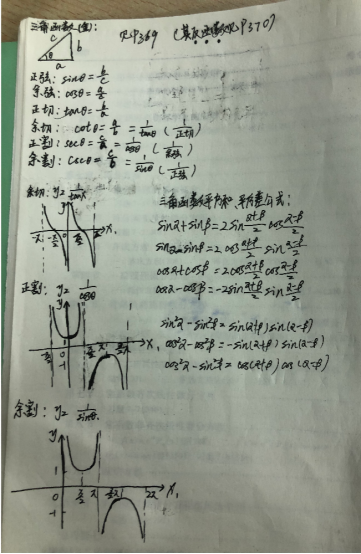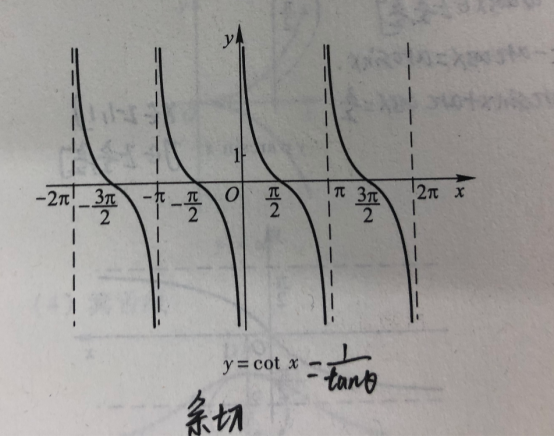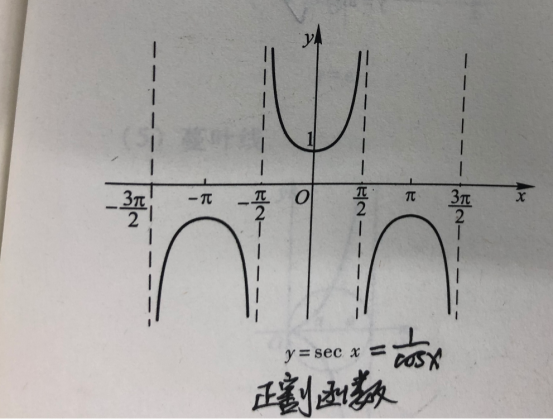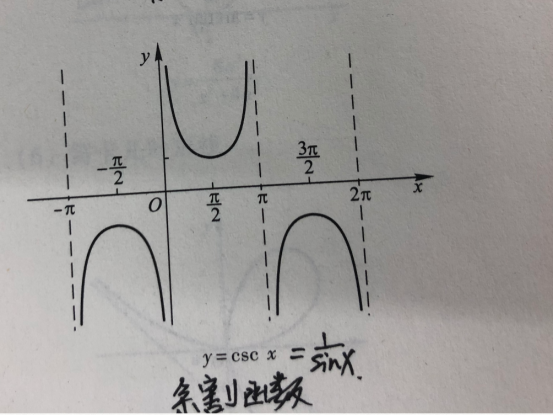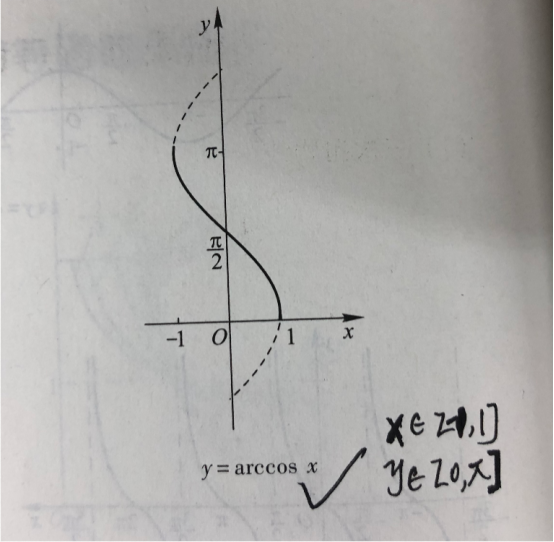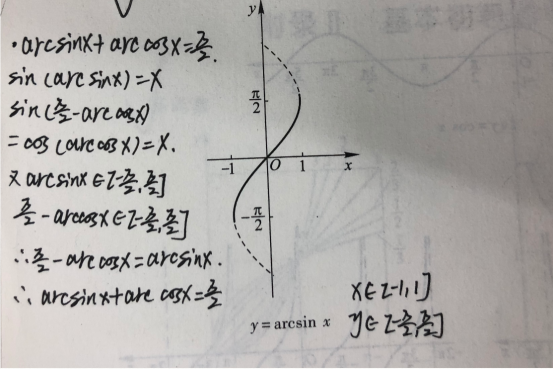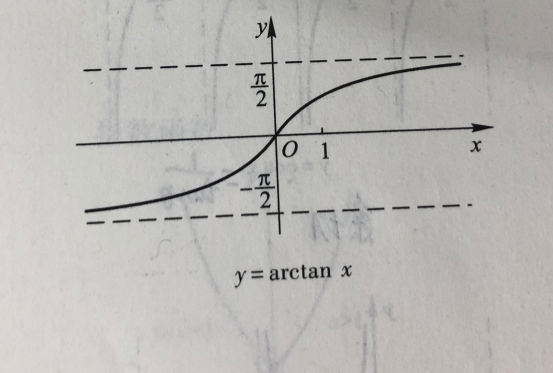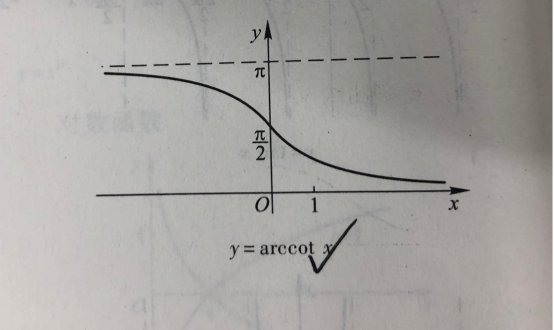展开全文• 16341019 数据科学与...三角函数公式 反三角函数公式 简单函数图像1三角函数公式两角和公式 sin(A+B) = sinAcosB+cosAsinBsin(A-B) = sinAcosB-cosAsinBcos(A+B) = cosAcosB-sinAsinBcos(A-B) = cosAcosB+sinAs
• 这些是修正的正弦、余弦、正切、割线、余割和余切函数，它们以度为单位进行论证。 它们会自动将度数转换为弧度，如果您以弧度为单位传递值，则返回值。matlab
• 第一章 第节 利用三角函数测高练习题及答案解析精选.doc
• 三角函数转换公式1、诱导公式：sin(-α)= -sinα；cos(-α) = cosα；sin(π/2-α)= cosα；cos(π/2-α) = sinα； sin(π/2+α) = cosα；cos(π/2+α)= -sinα；sin(π-α) = sinα；cos(π-α) = -cosα； sin...
• 三角函数计算器是一款非常小巧的计算机软件，能够让用户超级轻松的计算三角函数，对三角形的角度、边长进行计算，使用起来非常的方便快捷，大大节省大家的时间，赶紧来看看吧！三角函数计算器简介三角函数计算器是...
• 2019年沪教版高一第二学期第三角函数单元练习题.pdf
• 入读国际高中或就读美高的同学普遍三角函数(trigonometric function)学得不是很好，有些还停留在画三角形、按计算器才能计算sin、cos、tan的水平，很大原因是国外教材注重自我探究，通过一系列的循循善诱来给出结论...
• 三角函数计算器是一款绿色实用、安全稳定的计算小工具。它提供了六种基本函数计算，包括正弦、余弦、正切、余切等，能将计算结果精确到度、分、秒及自定义的小数位。由于三角函数的特殊性，软件不仅仅可以用于计算...
• 2017_2018学年高中数学第一章三角函数1.3三角函数的诱导公式第2课时三角函数的诱导公式五～优化练习新人教A版必修4201807314117
• 一、三角函数的概念单位圆定义：设起点在原点的射线，与x轴正半轴形成一个角θ，并与单位圆(x2+y2=1)相交。这个交点的横坐标值和纵坐标值分别等于cosθ和sinθ。单位圆定义允许三角函数对所有正数和负数辐角都有定义...
• 函数图像三角函数与反三角函数1.正弦函数 sin x， 反正弦函数 arcsin x2.余弦函数 cos x， 反余弦函数 arccos x3.反正弦函数 arcsin x， 反余弦函数 arccos x4.正切函数 tan x， 余切函数 cot x5.反正切函数 arctan ...
• 三角函数定义域y=arcsin(x)，定义域[-1，1]y=arccos(x)，定义域[-1，1]y=arctan(x)，定义域(-∞， ∞)y=arccot(x)，定义域(-∞， ∞)sin(arcsin x)=x，定义域[-1，1]反三角函数公式大全两角和公式sin(A B) = ...逆函数求导公式
• 上海市17区县2013届高三数学一模分类汇编 专题 三角函数
• ## 用python画三角函数

千次阅读 2020-12-04 19:52:12
Pyplothttp://www.labri.fr/perso/nrougier/teaching/matplotlib/pyplot提供了一个方便的matplotlib...简单的画图simple plot我们想在同一个图中划出一个cos和sin函数图，第一步是获取cos和sin的数据，构建函数imp...
• 三角函数 正弦sin 余弦cos 正切tan 余切cot 正割sec 余割csc 换算速记 1、倒数关系 tanα ·cotα＝1 ；sinα ·cscα＝1 ；cosα ·secα＝1 2、商数乘积关系 tanα=sinα/cosα ；cotα=cosα/sinα，tanθ=sin...
• 【名师导学】（新课标）2015高考数学一轮巩固 第三角函数的概念及三角变换同步检测课件 文
• 高中数学诱导公式全集常用的诱导公式有以下几组： 公式一：设α为任意角，终边相同的角的同一三角函数的...(k∈Z)公式二：设α为任意角，π+α的三角函数值与α的三角函数值之间的关系：　sin(π＋α)＝－sinα　cos...
• 解释：奇变偶不变，符号看象限对于kπ/2±α(k∈Z)的三角函数值，①当k是偶数时，得到α的同名函数值，即函数名不改变；②当k是奇数时，得到α相应的余函数值，即sin→cos;cos→sin;tan→cot,cot→tan.(奇变偶不变)...
• 九年级数学同步培优竞赛详附答案16第十讲锐角三角函数.pdf
• 之前对三角函数的理解仅局限于sin，cos，tan。但是目前遇到的都是些csc，sec，cot，arctan，arccos，arcsin。积分和求导还有一堆公式 最近看到了一个六边形记忆法，更加简便。
• 2019_2020学年高中数学第1章三角函数1.3三角函数的诱导公式第2课时诱导公式五课件新人教A版必修4202004270540
• 2019_2020学年高中数学第1章三角函数1.3三角函数的诱导公式第2课时诱导公式五练习新人教A版必修420200427057
• E201_06_02_正弦函数题目要求：按照三角函数泰勒级数展开式计算正弦函数值：,直到最后一项的绝对值小于106解题思路：1.输入弧度2.确定初始化值3.求阶梯函数代码：public class E201_06_02_正弦函数 {public static ......